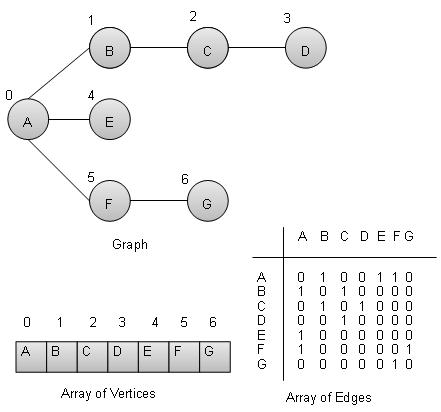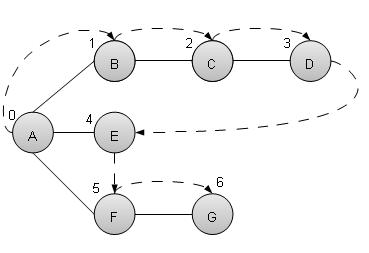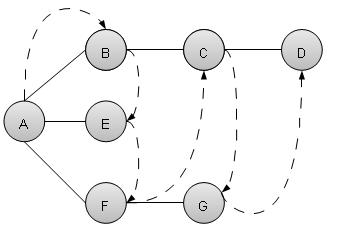# DSA using C - Graph

## Overview

Graph is a datastructure to model the mathematical graphs. It consists of a set of connected pairs called edges of vertices. We can represent a graph using an array of vertices and a two dimentional array of edges.

Important terms

• Vertex − Each node of the graph is represented as a vertex. In example given below, labeled circle represents vertices. So A to G are vertices. We can represent them using an array as shown in image below. Here A can be identified by index 0. B can be identified using index 1 and so on.

• Edge − Edge represents a path between two vertices or a line between two vertices. In example given below, lines from A to B, B to C and so on represents edges. We can use a two dimentional array to represent array as shown in image below. Here AB can be represented as 1 at row 0, column 1, BC as 1 at row 1, column 2 and so on, keeping other combinations as 0.

• Adjacency − Two node or vertices are adjacent if they are connected to each other through an edge. In example given below, B is adjacent to A, C is adjacent to B and so on.

• Path − Path represents a sequence of edges between two vertices. In example given below, ABCD represents a path from A to D.## Basic Operations

Following are basic primary operations of a Graph which are following.

• Add Edge − add an edge between two vertices of a graph.

• Display Vertex − display a vertex of a graph.

```//add vertex to the vertex list
struct vertex* vertex =
(struct vertex*) malloc(sizeof(struct vertex));
vertex->label = label;
vertex->visited = false;
lstVertices[vertexCount++] = vertex;
}
```

```//add edge to edge array
}
```

## Display Edge Operation

```//display the vertex
void displayVertex(int vertexIndex){
printf("%c ",lstVertices[vertexIndex]->label);
}
```

## Traversal Algorithms

Following are important traversal algorithms on a Graph.

• Depth First Search − traverses a graph in depthwards motion.

## Depth First Search Algorithm

Depth First Search algorithm(DFS) traverses a graph in a depthward motion and uses a stack to remember to get the next vertex to start a search when a dead end occurs in any iteration.As in example given above, DFS algorithm traverses from A to B to C to D first then to E, then to F and lastly to G. It employs following rules.

• Rule 1 − Visit adjacent unvisited vertex. Mark it visited. Display it. Push it in a stack.

• Rule 2 − If no adjacent vertex found, pop up a vertex from stack. (It will pop up all the vertices from the stack which do not have adjacent vertices.)

• Rule 3 − Repeat Rule 1 and Rule 2 until stack is empty.

```void depthFirstSearch(){
int i;
//mark first node as visited
lstVertices->visited = true;

//display the vertex
displayVertex(0);

//push vertex index in stack
push(0);

while(!isStackEmpty()){
//get the unvisited vertex of vertex which is at top of the stack

if(unvisitedVertex == -1){
pop();
} else {
lstVertices[unvisitedVertex]->visited = true;
displayVertex(unvisitedVertex);
push(unvisitedVertex);
}
}
//stack is empty, search is complete, reset the visited flag
for(i=0;i < vertexCount;i++){
lstVertices[i]->visited = false;
}
}
```

Breadth First Search algorithm(BFS) traverses a graph in a breadthwards motion and uses a queue to remember to get the next vertex to start a search when a dead end occurs in any iteration.As in example given above, BFS algorithm traverses from A to B to E to F first then to C and G lastly to D. It employs following rules.

• Rule 1 − Visit adjacent unvisited vertex. Mark it visited. Display it. Insert it in a queue.

• Rule 2 − If no adjacent vertex found, remove the first vertex from queue.

• Rule 3 − Repeat Rule 1 and Rule 2 until queue is empty.

```void breadthFirstSearch(){
int i;
//mark first node as visited
lstVertices->visited = true;

//display the vertex
displayVertex(0);

//insert vertex index in queue
insert(0);
int unvisitedVertex;
while(!isQueueEmpty()){
//get the unvisited vertex of vertex which is at front of the queue
int tempVertex = removeData();

lstVertices[unvisitedVertex]->visited = true;
displayVertex(unvisitedVertex);
insert(unvisitedVertex);
}
}
//queue is empty, search is complete, reset the visited flag
for(i=0;i<vertexCount;i++){
lstVertices[i]->visited = false;
}
}
```

## Example

```#include <stdio.h>
#include <string.h>
#include <stdlib.h>
#include <stdbool.h>
#define MAX 10

struct Vertex {
char label;
bool visited;
};

//stack variables
int stack[MAX];
int top=-1;

//queue variables
int queue[MAX];
int rear=-1;
int front=0;
int queueItemCount = 0;

//graph variables
//array of vertices
struct Vertex* lstVertices[MAX];

//vertex count
int vertexCount = 0;

//stack functions
void push(int item) {
stack[++top]=item;
}
int pop() {
return stack[top--];
}
int peek() {
return stack[top];
}
bool isStackEmpty(){
}
//queue functions
void insert(int data){
queue[++rear] = data;
queueItemCount++;
}
int removeData(){
queueItemCount--;
return queue[front++];
}
bool isQueueEmpty(){
return queueItemCount == 0;
}
//graph functions
//add vertex to the vertex list
struct Vertex* vertex = (struct Vertex*) malloc(sizeof(struct Vertex));
vertex->label = label;
vertex->visited = false;
lstVertices[vertexCount++] = vertex;
}
}
//display the vertex
void displayVertex(int vertexIndex){
printf("%c ",lstVertices[vertexIndex]->label);
}
int i;
for(i=0; i<vertexCount; i++)
return i;
return -1;
}
void depthFirstSearch(){
int i;
//mark first node as visited
lstVertices->visited = true;

//display the vertex
displayVertex(0);

//push vertex index in stack
push(0);

while(!isStackEmpty()){
//get the unvisited vertex of vertex which is at top of the stack

if(unvisitedVertex == -1){
pop();
} else {
lstVertices[unvisitedVertex]->visited = true;
displayVertex(unvisitedVertex);
push(unvisitedVertex);
}
}
//stack is empty, search is complete, reset the visited flag
for(i=0;i < vertexCount;i++){
lstVertices[i]->visited = false;
}
}
int i;
//mark first node as visited
lstVertices->visited = true;

//display the vertex
displayVertex(0);

//insert vertex index in queue
insert(0);
int unvisitedVertex;
while(!isQueueEmpty()){
//get the unvisited vertex of vertex which is at front of the queue
int tempVertex = removeData();

lstVertices[unvisitedVertex]->visited = true;
displayVertex(unvisitedVertex);
insert(unvisitedVertex);
}
}
//queue is empty, search is complete, reset the visited flag
for(i=0;i<vertexCount;i++){
lstVertices[i]->visited = false;
}
}
main() {
int i, j;

for(i=0; i<MAX; i++) // set adjacency
for(j=0; j<MAX; j++) // matrix to 0

/*      1  2  3
* 0  |--B--C--D
* A--|
* |
* |     4
* |-----E
* |     5  6
* |  |--F--G
* |--|
*/
printf("Depth First Search: ");

//A B C D E F G
depthFirstSearch();

//A B E F C G D
}
```

If we compile and run the above program then it would produce following result −

```Depth First Search: A B C D E F G
Breadth First Search: A B E F C G D
```